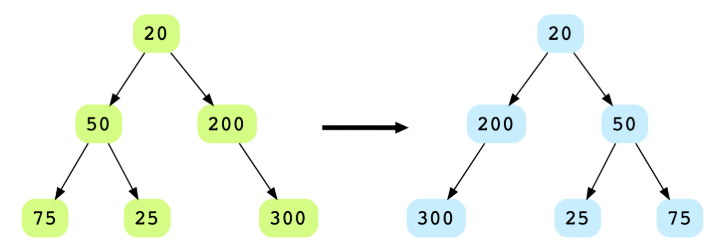# Mirror Binary Tree Nodes

## Problem Statement

Given the root node of a binary tree, swap the ‘left’ and ‘right’ children for each node. The below example shows how the mirrored binary tree should look like.## Hint

• Depth first traversal
• Bottom up mirroring

## Try it yourself

void mirror_tree(BinaryTreeNode* root) {  //TODO: Write - Your - Code}

## Solution

void mirror_tree(BinaryTreeNode* root) {  if (root == nullptr) {    return;  }  // We will do a post-order traversal of the binary tree.  if (root->left != nullptr) {    mirror_tree(root->left);  }  if (root->right != nullptr) {    mirror_tree(root->right);  }  // Let's swap the left and right nodes at current level.  BinaryTreeNode* temp = root->left;  root->left = root->right;  root->right = temp;}int main(int argc, char* argv[]) {  BinaryTreeNode* root = create_random_BST(15);  display_level_order(root);  mirror_tree(root);  cout << endl << "Mirrored tree = " << endl;  display_level_order(root);}

## Solution Explanation

### Runtime Complexity

Linear, O(n).

Every sub-tree needs to be mirrored so we visit every node once and mirror the sub-tree starting there. Hence run time complexity is O(n).

### Memory Complexity

Linear, O(n) in the worst case.

### Solution Breakdown

Recursive solution has O(h) memory complexity, for a balanced tree, as it will consume memory on the stack up to the height of the binary tree. For a skewed tree, it can be O(n).

Here, we will do a post order traversal of the binary tree. For every node, we will swap it’s left child with its right child. The original nodes are shown in green color while the nodes that have been swapped are shown in grey. The node at the top of the stack (current node) is shown in light blue. Note that we are doing DFS on the tree i.e. before returning from a node all its children have been visited (and mirrored).

Practice problems like this and many more by checking out our Grokking the Coding Interview: Patterns for Coding Questions course!

Learn in-demand tech skills in half the time